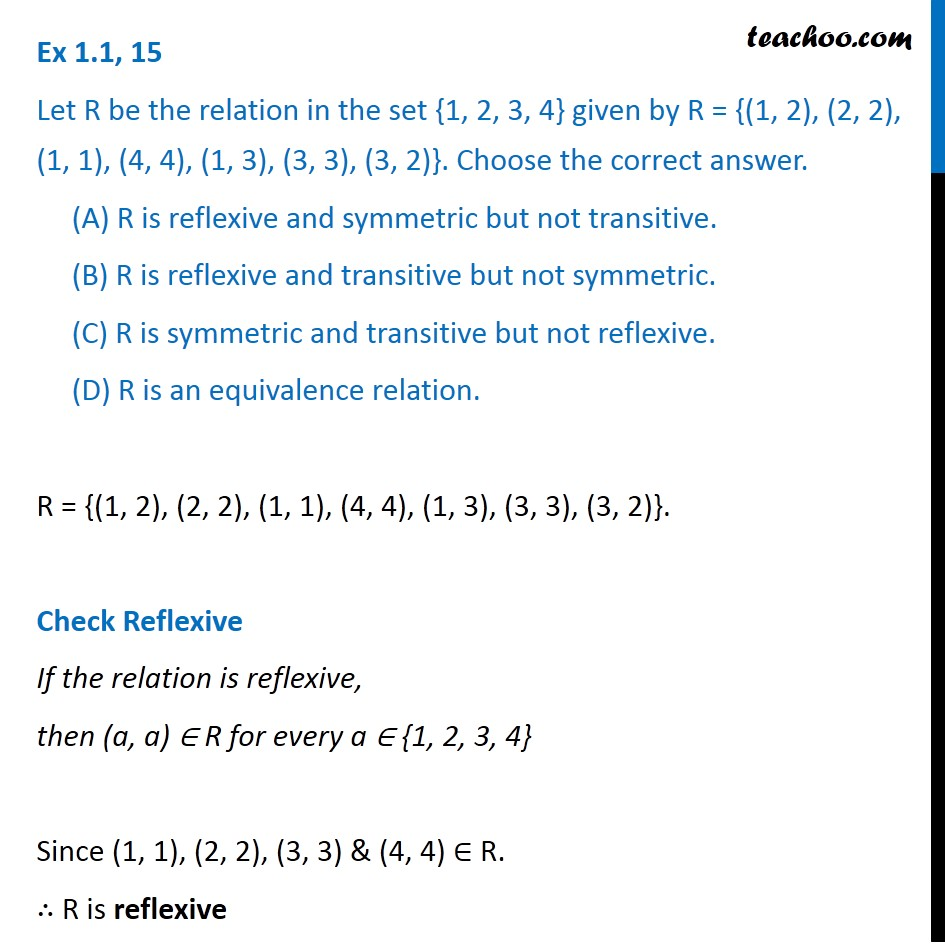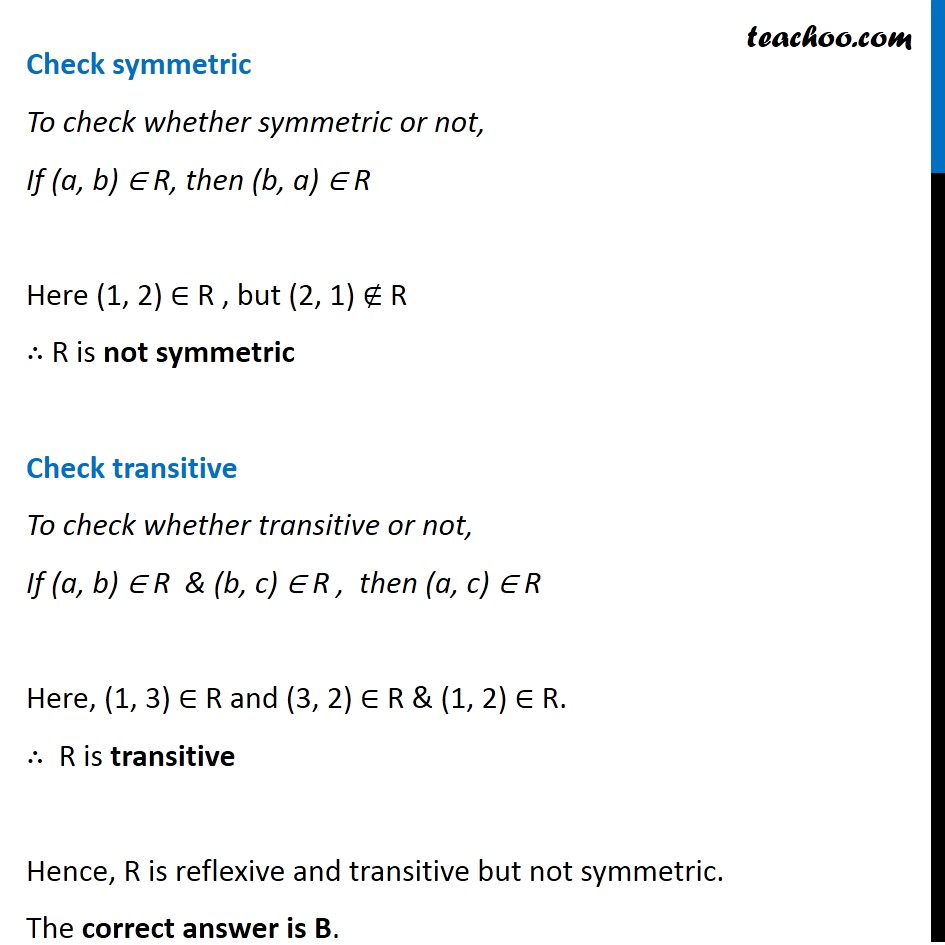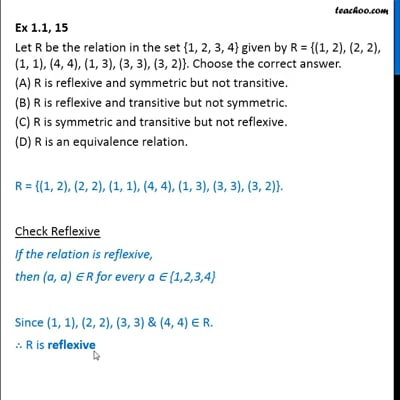To prove relation reflexive, transitive, symmetric and equivalent

Chapter 1 Class 12 Relation and Functions
Concept wiseThis video is only available for Teachoo black users

Introducing your new favourite teacher - Teachoo Black, at only ₹83 per month

### Transcript

Ex 1.1, 15 Let R be the relation in the set {1, 2, 3, 4} given by R = {(1, 2), (2, 2), (1, 1), (4, 4), (1, 3), (3, 3), (3, 2)}. Choose the correct answer. (A) R is reflexive and symmetric but not transitive. (B) R is reflexive and transitive but not symmetric. (C) R is symmetric and transitive but not reflexive. (D) R is an equivalence relation. R = {(1, 2), (2, 2), (1, 1), (4, 4), (1, 3), (3, 3), (3, 2)}. Check Reflexive If the relation is reflexive, then (a, a) ∈ R for every a ∈ {1, 2, 3, 4} Since (1, 1), (2, 2), (3, 3) & (4, 4) ∈ R. ∴ R is reflexive Check symmetric To check whether symmetric or not, If (a, b) ∈ R, then (b, a) ∈ R Here (1, 2) ∈ R , but (2, 1) ∉ R ∴ R is not symmetric Check transitive To check whether transitive or not, If (a, b) ∈ R & (b, c) ∈ R , then (a, c) ∈ R Here, (1, 3) ∈ R and (3, 2) ∈ R & (1, 2) ∈ R. ∴ R is transitive Hence, R is reflexive and transitive but not symmetric. The correct answer is B.